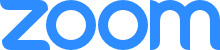#2021 SLA- Math 215 - Shared screen with speaker viewAshley Crockett
19:46
I am not able to hear to you Im not sure if its my computer or notJulius Ngwa
38:03
Positive Relationship between the Size and Price of the HouseAshley Crockett
38:15
noJulius Ngwa
38:28
Calculate the CorrelationJulius Ngwa
39:38
a = SlopeJulius Ngwa
39:42
b = InterceptJulius Ngwa
39:49
r and r-squareJulius Ngwa
39:57
Diagnostics are OFFJulius Ngwa
42:05
a = .099Julius Ngwa
42:13
b = 160.194Julius Ngwa
42:21
r-square = 0.811Julius Ngwa
42:27
r = 0.901Julius Ngwa
42:42
y = ax + bJulius Ngwa
45:27
regression equationJulius Ngwa
47:38
Actual ValueJulius Ngwa
47:48
Size = 3028 sq ftJulius Ngwa
47:58
Price = \$469,000Julius Ngwa
48:20
Predict Size = 3028 sq ft how close that is to the Actual ValueJulius Ngwa
48:33
How well our Regression Equation is able to PredictAshley Crockett
58:27
noJulius Ngwa
01:15:20
y = ax + bJulius Ngwa
01:15:33
Value attached to the x is the SlopeJulius Ngwa
01:15:45
Standalone value is the y-interceptJulius Ngwa
01:15:49
y = ax + bJulius Ngwa
01:15:57
a = slopeJulius Ngwa
01:16:01
b = y-interceptJulius Ngwa
01:16:07
y = a + bxJulius Ngwa
01:16:17
a = Y-interceptJulius Ngwa
01:16:19
b = slopeAshley Crockett
01:20:16
290.510Ashley Crockett
01:22:29
noAshley Crockett
01:23:12
I have noneAshley Crockett
01:23:43
thank you